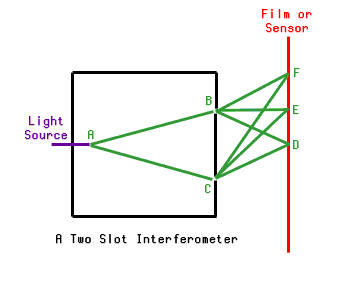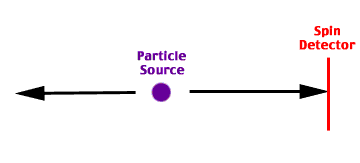# Divide by Psi

Robert Novella's Quantum Confusion paper raises the question of non-locality. Something which occurs at one point might in some sense "effect" something at some considerable distance. This is one of the paradoxical aspects of quantum mechanics, a problem which concerns main line physicists. As Novella suggests, the principle of non-locality is abused by pseudo scientists developing new age an psi theories.

This page explores non-locality in somewhat greater depth. It suggests a perspective by which non-locality might vanish. Non-locality is an artifact of the Copenhagen Convention that is not necessary, that can be eliminated by a change in perspectives and definitions.Again, the light source is very dim, so dim that the light recorded on the film (shown in red, on the right) shows up as single points of light, as individual photons. The light source is placed inside a box, a box with two slits at B and C. Photons are more likely to be observed at or near points D and F, where there are multiple paths such that the photon will arrive at the same point at the same phase. Photons are not apt to be observed at or near point B, where the two paths result in photons arriving exactly opposite in phase.

The above can be readily and repeatedly be observed, given a dim enough light source, sensitive enough film, and a really good dark room. The above is non-controversial, though not intuitive to those familiar only with Newton's every day causal view of reality.

An additional aspect of the apparent paradox is that detecting which path the particle took eliminates the interference effect. For example, suppose at point B, one placed a detector that senses any particles passing through slot B. If such a detector is placed and operated, the interference patterns vanish. The vicinity of point E is no longer particularly dark. F and D are no longer particularly light.

How does one explain this observed experimental result? By observing the particle at point B, one is inducing a "collapse" of the wave equation (using Copenhagen Convention terminology) or causing a split between now quite different Many Worlds realities. The alternate realities where the particle is detected at B become distinctly different from the realities where the particle was not detected. If multiple sets of realities are different, they do not interfere with one another. Once an observation has been made, the alternate universes where that observation has been made can not interact with any of the realities where different observations have been made.

This an example of how taking a measurement effects the system. In an abstract principle, one cannot observe a system without in some way disturbing it. When observing individual particle quantum interactions, the abstract principle becomes far more concrete. Only essentially identical alternate realities of the Many Worlds only interact with one another. Every time one takes a measurement, one is causing a branch in the Many Worlds alternate time lines. Placing a photon detector at B assures that for every particle that might pass through B, there is an alternate reality distinct from where the same particle passes through C. Given no detectors at B or C, the possibilities are uncollapsed, the alternate realities interfere.

But there is a distinction between whether the system is "collapsed" and whether the scientist running the system is yet aware of whether a collapse has occurred. Let us suppose that the detector at B is recording the times of particles passing through the slot onto a hard disk. Let us suppose that the film will not be developed for some time after the experiment has run. A collapse occurs when the detector at B goes off, or when the photon induces chemical changes in the film. However, it might be quite some time before the scientist examines the data and learns which of the Many Worlds he is occupying.

This can be a source of confusion. It is essential to the difference between Classic Copenhagen Convention and Neo Copenhagen Convention. Originally, it was the observation of a sentient being that collapsed the equation. With Neo-Copenhagen, the collapse is induced as soon as the action of the particle effects multiple other particles, regardless of whether sentience is involved.

Keeping that distinction in mind, we switch to non-locality.There are certain particles that decay into a pair of particles. The two new particles - obeying conservation laws - will travel in opposite directions, and have opposite spin. The process is in part random. There are multiple possible outcomes of the experiment. However, if one measures one particle, one learns things about the opposite particle. In this case, if one measure the spin of the right hand particle when it hits the detector, one instantly knows the spin of the other particle is opposite.

By some interpretations of quantum theory, the observation made of the right hand particle when it hits the spin detector instantly changes the state of the left hand particle. This is non-locality.

When does the collapse occur? If the collapse occurs when the two particles are emitted, there is no action at a distance, no instantaneous state change at a considerable distance from where the measurement was taken. If the state change only occurs when the measurement is taken, then one has the serious problem of instantaneous action at a distance.

Again, the distinction between physical collapse, a split in Many Worlds time lines, and the state change from ignorant to knowing in the sentient observer, should not be confused. The scientists definitely do not know the spin of the particle until it hits the detector. He cannot discard the alternate solutions to his problem until the data has been examined. However - in "reality" as opposed to the scientists mind - when does the spin get determined? Do particles moving right with positive spin interfere with particles moving right with negative spin? Or are particles with opposing spin in distinctly different realities, distinct enough that interaction is impossible?

If the latter, the problem of non-locality vanishes. Can one conceive of an experiment to answer the question empirically? I cannot.

What implications for psi? First, Novella is correct that pseudo scientists have overused the principle of non-locality. Non-locality says that if you measure one particle, and that you know it was produced as part of a pair, one also knows something about a second particle. It does not imply that the universe is one, or that all minds are interconnected. It is possible to interpret the wave equations as taking a measurement of one particle collapses a second particle, instantly, and at a distance. It might also be possible to interpret a measurement of one particle as telling one significant information on the particle's origin, at a time in the past. From that knowledge of the past, additional information about the paired particles' state in the present can be deduced.

Non-locality could be a mind trap, a delusion of no significance, an unintended and undesirable side effect of older variants of the Copenhagen Convention.

Non-locality might not even be firmly demonstrated. The principle of non-locality was first proposed as Bell's Theorem. Later, and experimental demonstration of Bell's Theorem was implemented. The first paper establishing the "proof" described a complex experimental set up, and the results of a half dozen trial particle pairs. There were more "proof" pairs than "disproof" pairs, but not every pair confirmed the theorem.

If the experimental "proof" of Bell's Theorem had been presented as a proof of psi, it would never have been published. There were too few samples taken, the statistical deviation from norm was not significant. This is an example of the double standards in science. If one's theory is an extension of the main line physical systems, a confirmation of something predicted by the traditional equations, the thinnest of proofs is accepted. This even holds if one is presenting a paper on a subject as controversial and important as non-locality. Non-locality is accepted on the thinnest of proofs, but the much more extensive psi data base is ignored.

There is a distinction between the equations and the interpretation of the equations. Non-locality is an interpretation. There are other possible interpretations. Applying these interpretations when one can't understand the equations is questionable. However, one might be careful even of the equations.

The quantum wave equations were originally developed to handle single particle systems. For quite some time, no one could solve them for multiple particle systems. The more one worked on the equations, the more complex the interactions became.

Physicist Richard Feynman - as was his wont - adapted a non-conventional solution. He divided both sides of the equations by a term that could reasonably hold the value zero. This is a no-no. This broke the rules. However, his solutions were meaningful. As solving the equations legitimately was impossible, physicists have ever since been breaking the rules, taking a shortcut.

But let's take a look at retrocausality? If retrocausality as suggested by Proving Murphy's Law is true, one would have to examine every possible future before determining the odds of what might happen in the present. Thus, if retrocausality is fact, one would expect that the basic quantum wave equations would be inherently unsolvable.

When Feynman divided out his possibly zero term, did he divide out retrocausality and psi?

Thus, one must both agree and disagree with Quantum Confusion. Taking assorted paradox and interpretation from quantum physics and applying them haphazardly to non-quantum systems is an invitation to self delusion. If one doesn't really understand the equations and the phenomenon that the equations explain, applying the principles and terminology to non-quantum systems is pseudo-science.

On the other hand, quantum mechanics contains controversy and paradox. It is a wonderfully descriptive system, the best system for explaining small scale phenomena, but it is not a finished system. Long term, it is going to fall. The best of its features will be contained in something more inclusive.

Will this new system include retrocausality, psi and metabolic feedback? Possibly. In the form described in Proving Murphy's Law? Maybe not. However, it is clear that to move on somebody has to think outside the box. If nothing else, Proving Murphy's Law is outside the box.Proving Murphy's Law